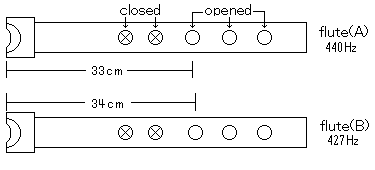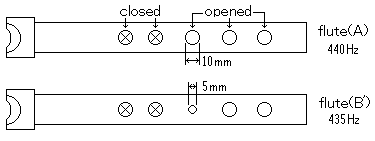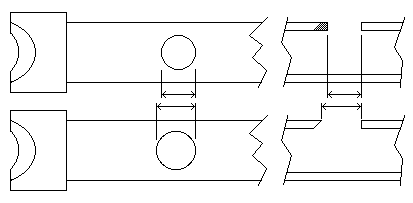## Tuning (ajusting the holes)

When all the holes are closed, the length of the bamboo flute is proportionate to the wave length of the sound. If the holes are opened, the distance from the mouth piece to the nearest opened hole is proportionate to the wave length of the sound, and then it is inversely proportionate to the frequency of the sound.
The shorter the distance from the mouth piece to the hole, the higher the frequency of the sound. For example as shown in the picture below, the sound of flute(A) which is 33cm from the hole can make a 440hz frequency sound. If you make a hole 1cm farther down than flute(A)'s hole like that of flute(B), you can get lower frequent sound.

the frequency of flute(A) sound = 440hz
the frequency of flute(B) sound = 440hz x (33/34) = almost 427hzThe smaller hole( flute B') will make lower frequent sound.If the sound frequency is lower than you aimed, you don't need another pipe. You can make the frequency higher by only widening a hole as shown in the picture below. But you can't make the frequency lower by adjusting the hole. You must take another PCB pipe and make a smaller hole at a farther position for lower frequency sound.For correct tuning of the flute (correct adjustment of the hole), you must know the frequency of the sound. You can get the sound frequency easily using the "shakuhachi tuning meter".